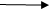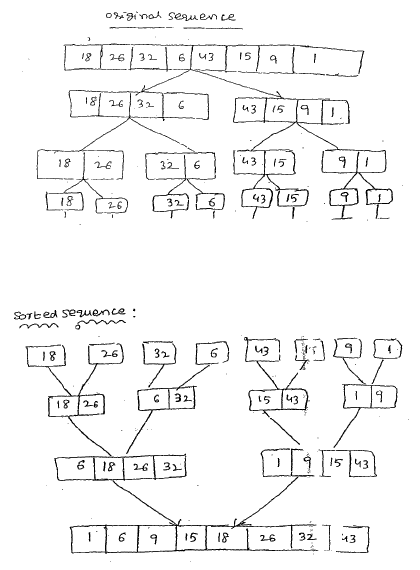# Explain about Merge Sort in DataStructures

12 September, 2018

Ratings

#### Related Blogs

Merge Sort

• Merging a two lists of one element each is the same as sorting them.
• Merge sort divides up an unsorted list until the above condition is met and then sorts the divided parts back together in pairs.
• Specifically this can be done by recursively dividing the unsorted list in half, merge sorting .The right side then the left side and then merging the right and left back

Merge sort algorithm: Given a list L with a length K: If k==1the list is sorted else

• merger sort the left side( 0thru k/2)
• merge sort the right side (k/2+1 thru K)
• merge the right with the left side.### program: Implementation of merge sort

# include <stdio.h> #include <conio.h> void merge (int numbers [], int temp[], int left,  int mid ,  int right); void merge sort (int numbers[], int temp[], int array-size); void m-sort( int numbers[],int temp[],int left, int right); void merge-sort ( int numbers [],int temp, int array-size); { m-sort(numbers,temp,0,array-size-1); } void m-sort(int numbers[],int temp[],int left, int right) { int mid; if(right>left) { mid = (right +left)/2; m-sort (numbers,  temp, left,mid); m-sort(numbers temp,mid+1,right); merge(numbers,temp,left,mid+1,right); } /*merge*/ void merge(int numbers[],int temp[],int left, int mid, int right) { int i,left-end,num-elements,Emp-pos; left-end =mid-1; emp-pos-left; num-elements =right-left+1; while ((left<=left-end)&& (mid<=right)) { if(numbers[left]<=numbers[mid]) { temp[temp-pos]=numbers[left]; tmp-pos = tmp-pos+1; left=left+1; else {temp[tmp-pos]= numbers [mid]; tmp-pos=tmp-pos+1; mid=mid+1; } } while( left <= left-end) { temp (tmp-pos]= numbers[left]; left=left+1; tmp=pos=tmp=pos+1; } while(mid <=right) { temp[tmp-pos]= numbers[mid]; mid=mid+1; tmp-pos=tmp-pos+1; } for(i=0;i<=num-elements;i++) { numbers [right ]= temp[right]; right =right-1; } } void main() { int a,b,n,i; printf(“Enter the value of  n:\n”); scanf(“%d”,&n); printf(“Enter n elements :\n”); for (i=0;i<=n;i++) { scanf(“%d”,&a[i]); } merger sort(a,b,n); printf (“Sorted elements are :\n”); for (i=0;i<n;i++) { printf(“%d”,a[i]); } }         OUTPUT: Enter the value of n:8 Enter n elements: 18 26 32 6 43 15 9 1 sorted elements are: 1  6  9 15 18  26 32 43                         Example: